# The velocity-displacement graph of a particle is shown in the figure.

Question:

The velocity-displacement graph of a particle is shown in the figure.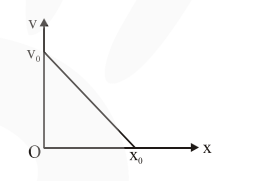The acceleration-displacement graph of the same particle is represented by :

1.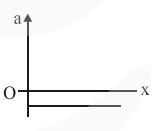2.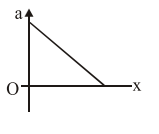3.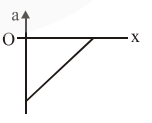4.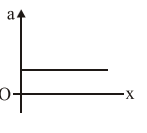Correct Option: , 3

Solution:

$\mathrm{V}=-\left(\frac{\mathrm{V}_{0}}{\mathrm{x}_{0}}\right) \mathrm{x}+\mathrm{v}_{0}$

$a=\frac{v d v}{d x}$

$a=\left[-\left(\frac{v_{0}}{x_{0}}\right) x+v_{0}\right]\left[-\frac{v_{0}}{x_{0}}\right]$

$\mathrm{a}=\left(\frac{\mathrm{v}_{0}}{\mathrm{x}_{0}}\right)^{2} \mathrm{x}-\frac{\mathrm{v}_{0}^{2}}{\mathrm{x}_{0}}$